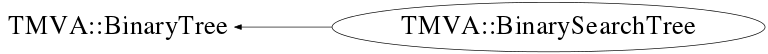# class TMVA::BinarySearchTree: public TMVA::BinaryTree

```
BinarySearchTree

A simple Binary search tree including a volume search method

```

## Function Members (Methods)

public:
 virtual ~BinarySearchTree() virtual void* TMVA::BinaryTree::AddXMLTo(void* parent) const TMVA::BinarySearchTree BinarySearchTree() TMVA::BinarySearchTree BinarySearchTree(const TMVA::BinarySearchTree& b) TMVA::BinaryTree TMVA::BinaryTree::BinaryTree() TMVA::BinaryTree TMVA::BinaryTree::BinaryTree(const TMVA::BinaryTree&) void CalcStatistics(TMVA::Node* n = 0) static TClass* Class() virtual const char* ClassName() const void Clear(TMVA::Node* n = 0) UInt_t TMVA::BinaryTree::CountNodes(TMVA::Node* n = __null) static TMVA::BinarySearchTree* CreateFromXML(void* node, UInt_t tmva_Version_Code = 262656) virtual TMVA::Node* CreateNode(UInt_t) const virtual TMVA::BinaryTree* CreateTree() const Double_t Fill(const vector& events, Int_t theType = -1) Double_t Fill(const vector& events, const vector& theVars, Int_t theType = -1) TMVA::Node* TMVA::BinaryTree::GetLeftDaughter(TMVA::Node* n) UInt_t TMVA::BinaryTree::GetNNodes() const UInt_t GetPeriode() const TMVA::Node* TMVA::BinaryTree::GetRightDaughter(TMVA::Node* n) virtual TMVA::Node* TMVA::BinaryTree::GetRoot() const Double_t GetSumOfWeights() const Double_t GetSumOfWeights(Int_t theType) const UInt_t TMVA::BinaryTree::GetTotalTreeDepth() const void Insert(const TMVA::Event*) virtual TClass* IsA() const Float_t Max(TMVA::Types::ESBType sb, UInt_t var) Float_t Mean(TMVA::Types::ESBType sb, UInt_t var) Float_t Min(TMVA::Types::ESBType sb, UInt_t var) void NormalizeTree() TMVA::BinarySearchTree& operator=(const TMVA::BinarySearchTree&) virtual void TMVA::BinaryTree::Print(ostream& os) const virtual void TMVA::BinaryTree::Read(istream& istr, UInt_t tmva_Version_Code = 262656) virtual void TMVA::BinaryTree::ReadXML(void* node, UInt_t tmva_Version_Code = 262656) Float_t RMS(UInt_t var) Float_t RMS(TMVA::Types::ESBType sb, UInt_t var) TMVA::BinarySearchTreeNode* Search(TMVA::Event* event) const Double_t SearchVolume(TMVA::Volume*, vector* events = 0) Int_t SearchVolumeWithMaxLimit(TMVA::Volume*, vector* events = 0, Int_t = -1) void SetNormalize(Bool_t norm) void SetPeriode(Int_t p) void TMVA::BinaryTree::SetRoot(TMVA::Node* r) void TMVA::BinaryTree::SetTotalTreeDepth(Int_t depth) void TMVA::BinaryTree::SetTotalTreeDepth(TMVA::Node* n = __null) virtual void ShowMembers(TMemberInspector& insp) const virtual void Streamer(TBuffer&) void StreamerNVirtual(TBuffer& ClassDef_StreamerNVirtual_b)
protected:
 void TMVA::BinaryTree::DeleteNode(TMVA::Node*) TMVA::MsgLogger& TMVA::BinaryTree::Log() const
private:
 void DestroyNode(TMVA::BinarySearchTreeNode*) void Insert(const TMVA::Event*, TMVA::Node*) Bool_t InVolume(const vector&, TMVA::Volume*) const void NormalizeTree(vector >::iterator, vector >::iterator, UInt_t) TMVA::BinarySearchTreeNode* Search(TMVA::Event*, TMVA::Node*) const Double_t SearchVolume(TMVA::Node*, TMVA::Volume*, Int_t, vector* events)

## Data Members

protected:
 UInt_t TMVA::BinaryTree::fDepth maximal depth in tree reached UInt_t TMVA::BinaryTree::fNNodes total number of nodes in the tree (counted) TMVA::Node* TMVA::BinaryTree::fRoot the root node of the tree
private:
 Bool_t fCanNormalize the tree can be normalised UInt_t fCurrentDepth internal variable, counting the depth of the tree during insertion vector fMax RMS for signal and background for each variable vector fMeans mean for signal and background for each variable vector fMin RMS for signal and background for each variable Double_t fNEventsW Number of events per class, taking into account event weights vector > fNormalizeTreeTable UInt_t fPeriod periode (number of event variables) vector fRMS RMS for signal and background for each variable Bool_t fStatisticsIsValid flag if last stat calculation is still valid, set to false if new node is insert vector fSum Sum for signal and background for each variable Double_t fSumOfWeights Total number of events (weigthed) counted during filling vector fSumSq Squared Sum for signal and background for each variable

## Class Charts## Function documentation

BinarySearchTree(const TMVA::BinarySearchTree& b)
``` default constructor
```
BinarySearchTree(const TMVA::BinarySearchTree& b)
``` copy constructor that creates a true copy, i.e. a completely independent tree
```
~BinarySearchTree( void )
``` destructor
```
TMVA::BinarySearchTree* CreateFromXML(void* node, UInt_t tmva_Version_Code = 262656)
``` re-create a new tree (decision tree or search tree) from XML
```
void Insert(const TMVA::Event* )
``` insert a new "event" in the binary tree
```
void Insert(const TMVA::Event* , TMVA::Node* )
``` private internal function to insert a event (node) at the proper position
```
TMVA::BinarySearchTreeNode* Search(TMVA::Event* event) const
```search the tree to find the node matching "event"
```
TMVA::BinarySearchTreeNode* Search(TMVA::Event* , TMVA::Node* ) const
``` Private, recursive, function for searching.
```
Double_t GetSumOfWeights(Int_t theType) const
```return the sum of event (node) weights
```
Double_t GetSumOfWeights(Int_t theType) const
```return the sum of event (node) weights
```
Double_t Fill(const vector<TMVA::Event*>& events, const vector<Int_t>& theVars, Int_t theType = -1)
``` create the search tree from the event collection
using ONLY the variables specified in "theVars"
```
Double_t Fill(const vector<TMVA::Event*>& events, Int_t theType = -1)
``` create the search tree from the events in a TTree
using ALL the variables specified included in the Event
```
void NormalizeTree(vector<pair<double,const TMVA::Event*> >::iterator , vector<pair<double,const TMVA::Event*> >::iterator , UInt_t )
void NormalizeTree()
``` Normalisation of tree
```
void Clear(TMVA::Node* n = 0)
``` clear nodes
```
Double_t SearchVolume(TMVA::Volume* , vector<const TMVA::BinarySearchTreeNode*>* events = 0)
``` search the whole tree and add up all weigths of events that
lie within the given voluem
```
Double_t SearchVolume(TMVA::Node* , TMVA::Volume* , Int_t , vector<const TMVA::BinarySearchTreeNode*>* events)
``` recursively walk through the daughter nodes and add up all weigths of events that
lie within the given volume
```
Bool_t InVolume(const vector<Float_t>& , TMVA::Volume* ) const
``` test if the data points are in the given volume
```
void CalcStatistics(TMVA::Node* n = 0)
``` calculate basic statistics (mean, rms for each variable)
```
Int_t SearchVolumeWithMaxLimit(TMVA::Volume* , vector<const TMVA::BinarySearchTreeNode*>* events = 0, Int_t = -1)
``` recursively walk through the daughter nodes and add up all weigths of events that
lie within the given volume a maximum number of events can be given
```
Node * CreateNode(UInt_t ) const
`{ return new BinarySearchTreeNode(); }`
BinaryTree* CreateTree() const
`{ return new BinarySearchTree(); }`
const char* ClassName() const
`{ return "BinarySearchTree"; }`
void SetPeriode(Int_t p)
```set the periode (number of variables)
```
`{ fPeriod = p; }`
UInt_t GetPeriode( void )
``` return periode (number of variables)
```
`{ return fPeriod; }`
Float_t Mean(TMVA::Types::ESBType sb, UInt_t var)
``` access to mean for signal and background for each variable
```
`{ return fMeans[sb==Types::kSignal?0:1][var]; }`
Float_t RMS(TMVA::Types::ESBType sb, UInt_t var)
``` access to RMS for signal and background for each variable
```
`{ return fRMS[sb==Types::kSignal?0:1][var]; }`
Float_t Min(TMVA::Types::ESBType sb, UInt_t var)
``` access to Minimum for signal and background for each variable
```
`{ return fMin[sb==Types::kSignal?0:1][var]; }`
Float_t Max(TMVA::Types::ESBType sb, UInt_t var)
``` access to Maximum for signal and background for each variable
```
`{ return fMax[sb==Types::kSignal?0:1][var]; }`
Float_t RMS(UInt_t var)
``` access to RMS for each variable
```
`{ return fRMS[var]; }`
void SetNormalize(Bool_t norm)
`{ fCanNormalize = norm; }`
void DestroyNode(TMVA::BinarySearchTreeNode* )